# SAT Math Multiple Choice Question 329: Answer and Explanation

### Test Information

Question: 329

14. It is given that sin A = k, where A is an angle measured in radians and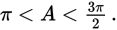If sin B = k, which of the following could be the value of B?

• A. A - π
• B. π + A
• C. 2π - A
• D. 3π - A

Explanation:

D

Difficulty: Hard

Category: Additional Topics in Math / Trigonometry

Strategic Advice: Try to determine how angles A and B are related, based on their corresponding sine values. Then, determine the quadrant in which angle B must lie, given the parameters of angle A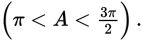Getting to the Answer: If an angle with measure A such that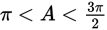is drawn on a unit circle, its terminal side will fall in Quadrant III, and sin A = k will be a negative value (because sine represents the y-value of the point that intersects the unit circle). If sin B = k also (and k is negative), then the terminal side of B must land in either of Quadrants III or IV (because sine is negative in those quadrants). Choose an easy radian measure (in Quadrant III) for angle A, such as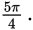Try each answer choice to see which one results in an angle that lies in the third or fourth quadrant: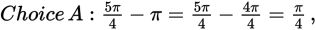which is in Quadrant I, so eliminate A.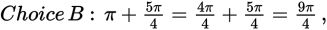which is in Quadrant I (because it is the same as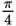rotated one full circle), so eliminate B.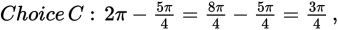which is in Quadrant II, so eliminate C.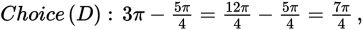which is in Quadrant IV, so (D) is correct.